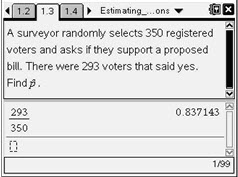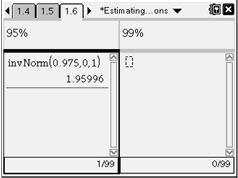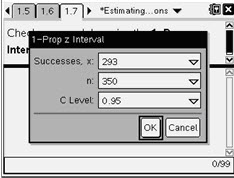# Activities

••• ##### Subject Area

• Math: Statistics: Statistical Inference

• ##### Author9-12

40 Minutes

• ##### Device
• TI-Nspire™
• TI-Nspire™ CAS
• ##### Software

TI-Nspire™
TI-Nspire™ CAS

2.0

## Estimating a Population Proportion#### Activity Overview

Students find the confidence interval for a population proportion by first finding the critical value and the margin of error. They find confidence intervals for real-life scenarios and use those intervals to make a judgment about a claim. Finally, they use two formulas for finding the required sample size for a survey, given a confidence interval and margin of error. The first formula assumes no estimate of the sample proportion, while the second one does.

#### Key Steps

•Students are to read page 1.3 and find the sample proportion by dividing. The sample proportion is about 0.837.

Note: For easy reference on the following pages in the tns file, students should store the proportion as p.

•On page 1.6, students are to find the critical value, the margin of error, and the intervals at both the 95% and 99% level.

•The handheld allows this confidence interval to be found without having to first find the critical value and margin of error. On page 1.7, students can check their work by pressing MENU > Statistics > Confidence Intervals > 1-Prop z Interval. Then, they enter x, n, and the confidence level (C Level).